### Sound and Acoustics: Sound Propagation

### Sound Propagation in One Dimension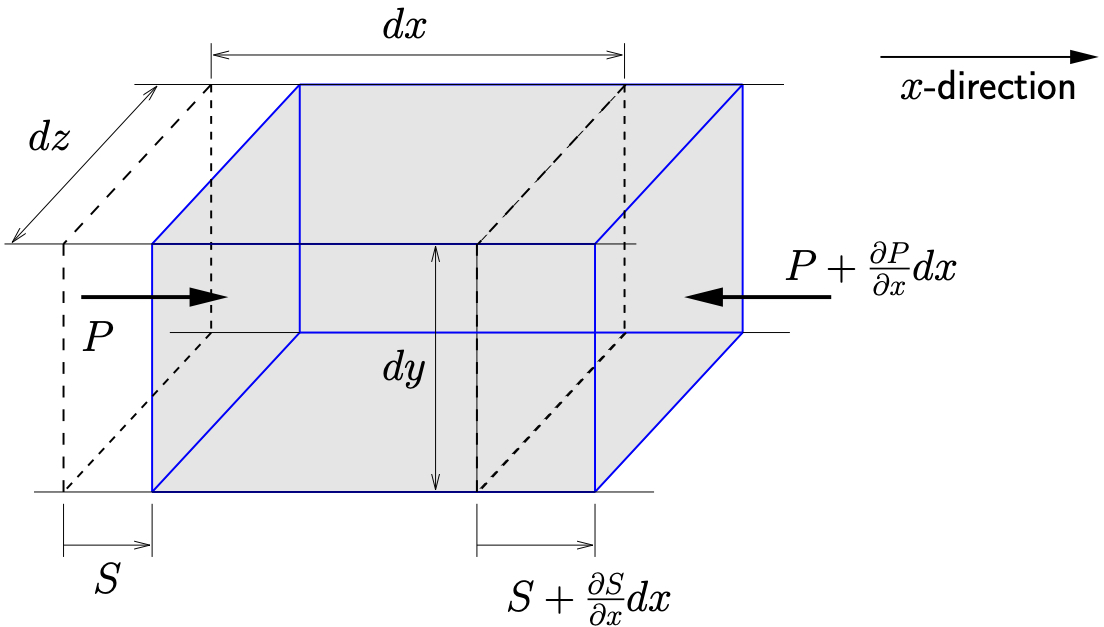Figure 12.1: Compression wave travelling in-direction

We begin by considering a longitudinal compression wave traveling in a fluid in the-direction. An infinitesimal element with initial dimensions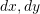and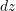is shown in Figure 12.1. This fluid element will deform as the wave passes and a deformed configuration of the fluid element is shown highlighted in gray. Here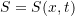represents the displacement of the fluid in the-direction while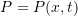is the acoustic overpressure in the fluid (\ie the pressure above the local atmospheric (equilibrium) pressure). At the left end of the deformed fluid element in Figure 12.1 the material has displaced an amount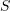and is acted upon by a pressure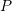. At the right end, the displacement and pressure are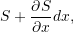and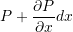respectively.

Application of Newton’s law in the-direction results in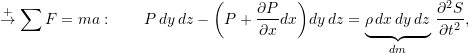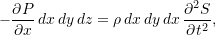or

(12.1)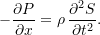We assume that the pressure (above atmospheric) is related to the volumetric strain by

(12.2)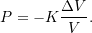where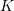is the bulk modulus of the fluid. Since the only motion of the fluid element is in the-direction, we see that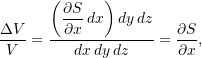so that 12.2 becomes

(12.3)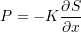and 12.1 becomes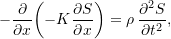or

(12.4)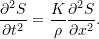This is the wave equation which governs the displacement of the fluid. To consider the response of the pressure, we begin by differentiating 12.1 with respect to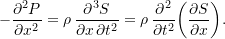Using the result from 12.3 this becomes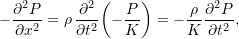which can be rearranged to give

(12.5)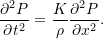Comparing 12.4 and 12.5 we see that both the displacement and the pressure satisfy the same wave equation. The wave speed in both cases is given by

(12.6)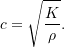This speed is known as the speed of sound in the medium.

### Speed of Sound

Generally in acoustics we are concerned with the transmission of sound through air. Under most conditions of interest, air can be treated as an ideal gas so that

(12.7)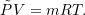Here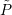is the total pressure of the air given by

(12.8)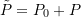where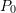is the atmospheric pressure andis the acoustic overpressure.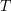in 12.7 is the absolute temperature and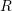is the ideal gas constant which for air is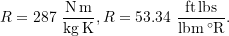#### Bulk Modulus

As we saw in 12.2, the change in pressure of a gas is related to the volumetric strain according to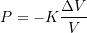which can be rearranged as

(12.9)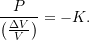Pressure fluctuations of this sort are an adiabatic (no heat transfer) process which for an ideal gas follow

(12.10)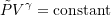where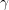is the ratio of specific heats (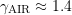). Differentiating 12.10 with respect to volume shows that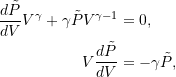or

(12.11)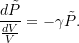Comparing 12.9 and 12.11 we see that for an ideal gas the bulk modulus is given by

(12.12)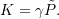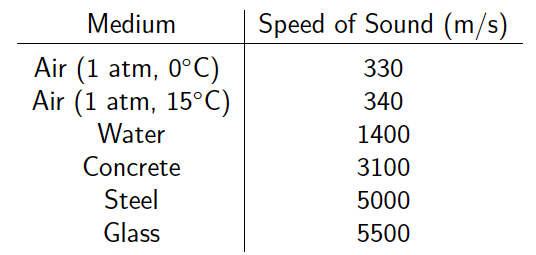Table 12.1: Typical speed of sound in various media

From 12.6 we know that the speed of sound is related to the bulk modulus and density by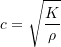which using 12.12 becomes

(12.13)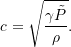However, from the ideal gas law we know that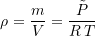so that 12.13 becomes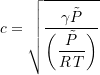or

(12.14)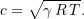With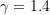and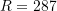, this gives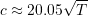in m/s whereis the absolute temperature.

For a solid, the bulk modulus of a material is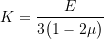where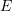is Young’s Modulus and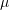is Poisson’s ratio. This represents how much a solid is compressed if acted upon by a hydrostatic stress state. Note that if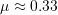, which is typical of many engineering materials, then the bulk modulus is approximately the same as Young’s Modulus. Table 12.1 shows the speed of sound in several media.

### Frequency and Wavelength

The speed of sound is related to \itspos frequency and wavelength by

(12.15)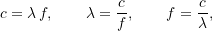where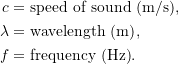Human hearing is nominally within the range of 20 Hz to 20 kHz. For air at 15 {°}C, (= 340 m/s) this corresponds to wavelengths ranging from 17 m to 17 mm.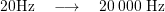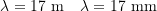Out of interest we can compare this to human vision. The visible light spectrum (= 3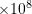m/s) nominally corresponds to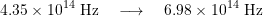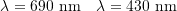Notice that for the visible light spectrum the ratio of the maximum frequency to the minimum frequency is less than two. For human hearing this ratio is about 1000.